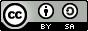# 数字识别¶

## 说明:¶

1. 硬件环境要求： 本文可支持在CPU、GPU下运行

2. Docker镜像支持的CUDA/cuDNN版本： 如果使用了Docker运行Book，请注意：这里所提供的默认镜像的GPU环境为 CUDA 8/cuDNN 5，对于NVIDIA Tesla V100等要求CUDA 9的 GPU，使用该镜像可能会运行失败。

3. 文档和脚本中代码的一致性问题： 请注意：为使本文更加易读易用，我们拆分、调整了train.py的代码并放入本文。本文中代码与train.py的运行结果一致，可直接运行train.py进行验证。

## 背景介绍¶MNIST数据集是从 NIST 的Special Database 3（SD-3）和Special Database 1（SD-1）构建而来。由于SD-3是由美国人口调查局的员工进行标注，SD-1是由美国高中生进行标注，因此SD-3比SD-1更干净也更容易识别。Yann LeCun等人从SD-1和SD-3中各取一半作为MNIST的训练集（60000条数据）和测试集（10000条数据），其中训练集来自250位不同的标注员，此外还保证了训练集和测试集的标注员是不完全相同的。

MNIST吸引了大量的科学家基于此数据集训练模型，1998年，LeCun分别用单层线性分类器、多层感知器（Multilayer Perceptron, MLP）和多层卷积神经网络LeNet进行实验，使得测试集上的误差不断下降（从12%下降到0.7%）。在研究过程中，LeCun提出了卷积神经网络（Convolutional Neural Network），大幅度地提高了手写字符的识别能力，也因此成为了深度学习领域的奠基人之一。此后，科学家们又基于K近邻（K-Nearest Neighbors）算法、支持向量机（SVM）、神经网络[4-7]和Boosting方法等做了大量实验，并采用多种预处理方法（如去除歪曲、去噪、模糊等）来提高识别的准确率。

## 模型概览¶

• $X$是输入：MNIST图片是$28\times28$ 的二维图像，为了进行计算，我们将其转化为$784$维向量，即$X=\left ( x_0, x_1, \dots, x_{783} \right )$。

• $Y$是输出：分类器的输出是10类数字（0-9），即$Y=\left ( y_0, y_1, \dots, y_9 \right )$，每一维$y_i$代表图片分类为第$i$类数字的概率。

• $Label$是图片的真实标签：$Label=\left ( l_0, l_1, \dots, l_9 \right )$也是10维，但只有一维为1，其他都为0。例如某张图片上的数字为2，则它的标签为$(0,0,1,0, \dots, 0)$

### Softmax回归(Softmax Regression)¶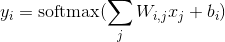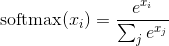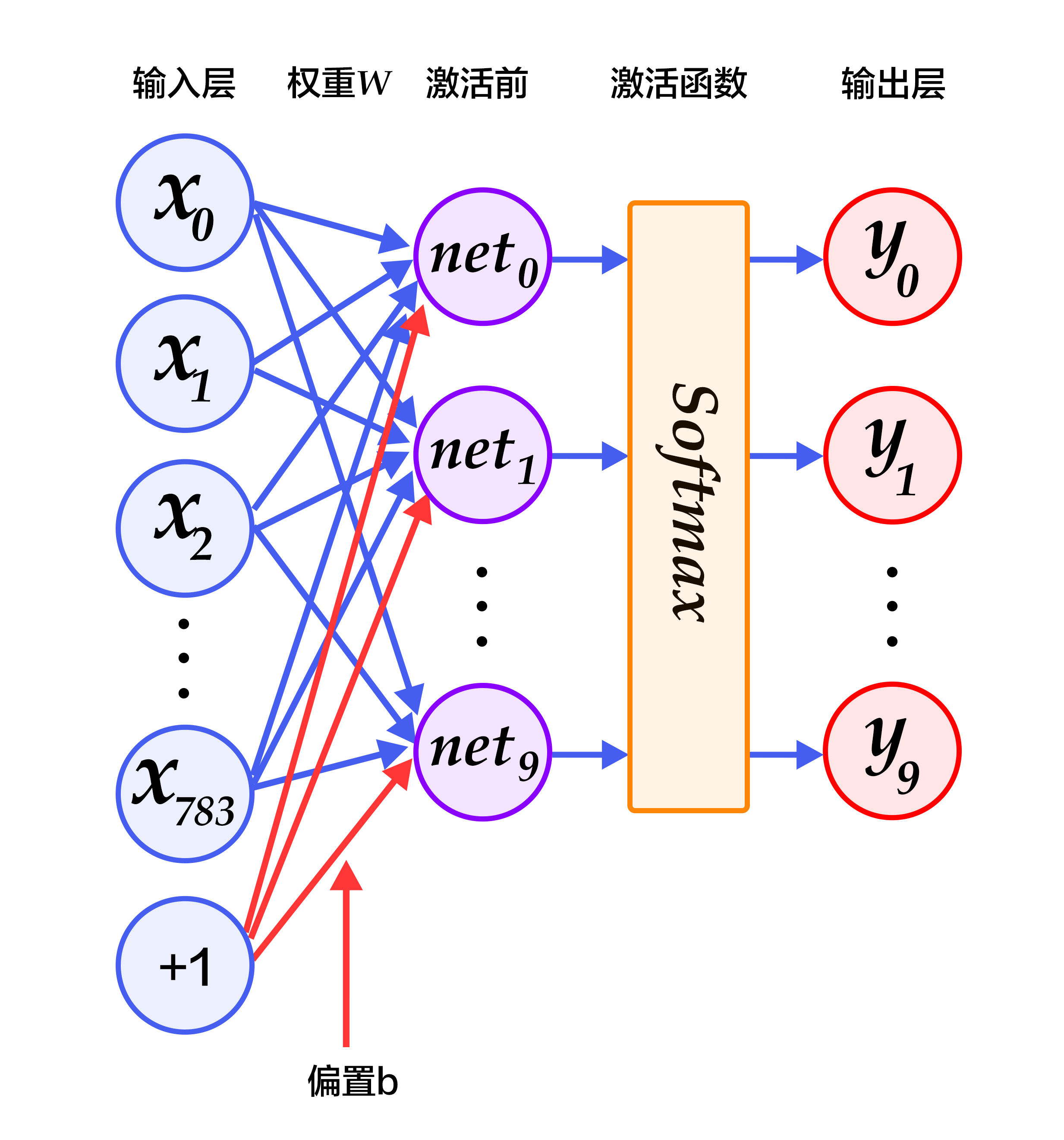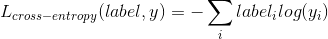### 多层感知机(Multilayer Perceptron, MLP)¶

Softmax回归模型采用了最简单的两层神经网络，即只有输入层和输出层，因此其拟合能力有限。为了达到更好的识别效果，我们考虑在输入层和输出层中间加上若干个隐藏层。

1. 经过第一个隐藏层，可以得到 $H_1 = \phi(W_1X + b_1)$，其中$\phi$代表激活函数，常见的有sigmoid、tanh或ReLU等函数。

2. 经过第二个隐藏层，可以得到 $H_2 = \phi(W_2H_1 + b_2)$。

3. 最后，再经过输出层，得到的$Y=\text{softmax}(W_3H_2 + b_3)$，即为最后的分类结果向量。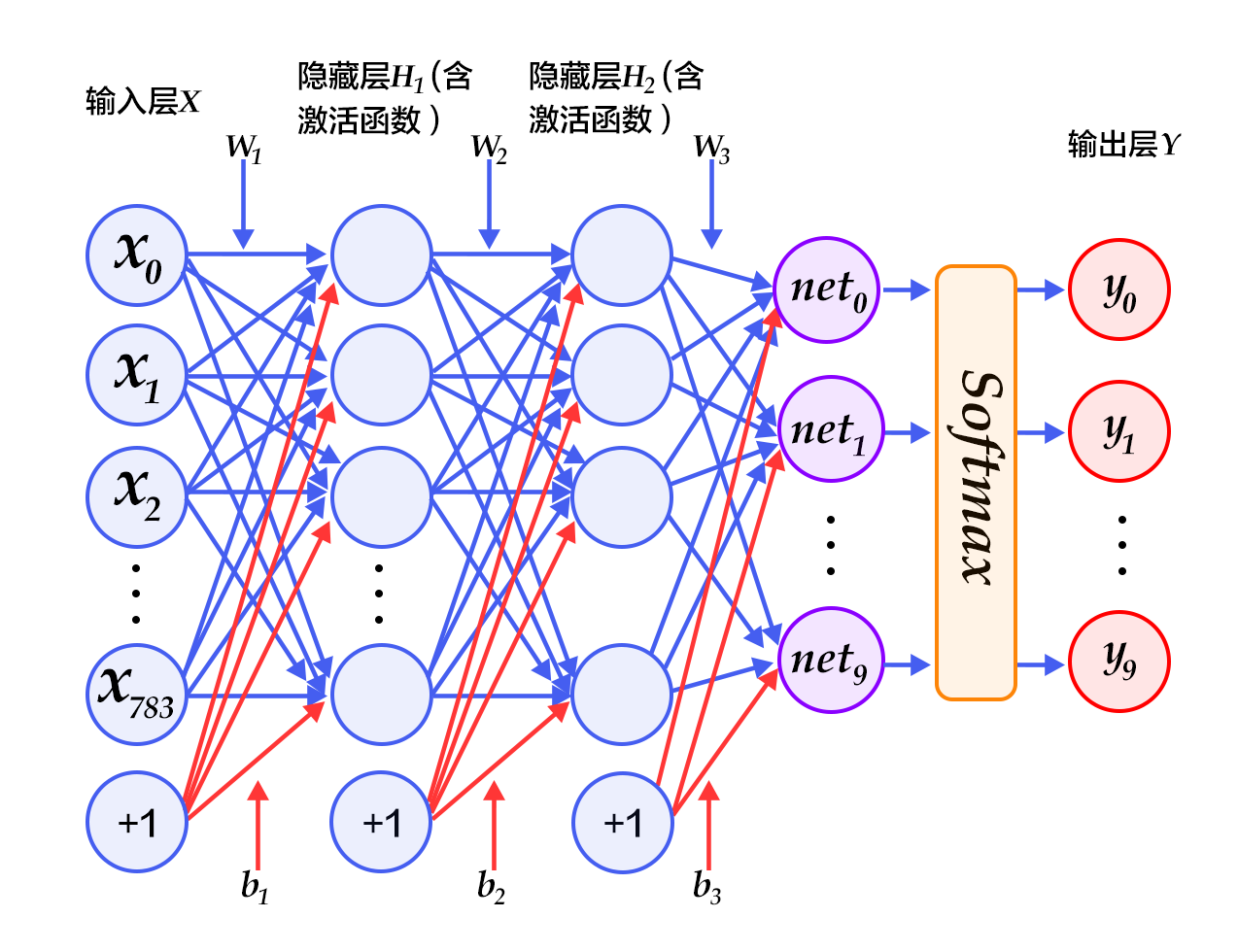### 卷积神经网络(Convolutional Neural Network, CNN)¶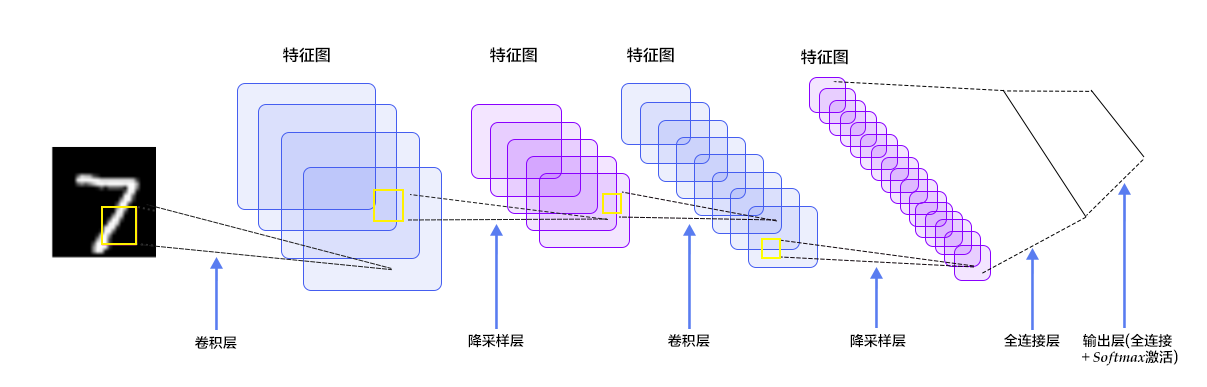#### 卷积层¶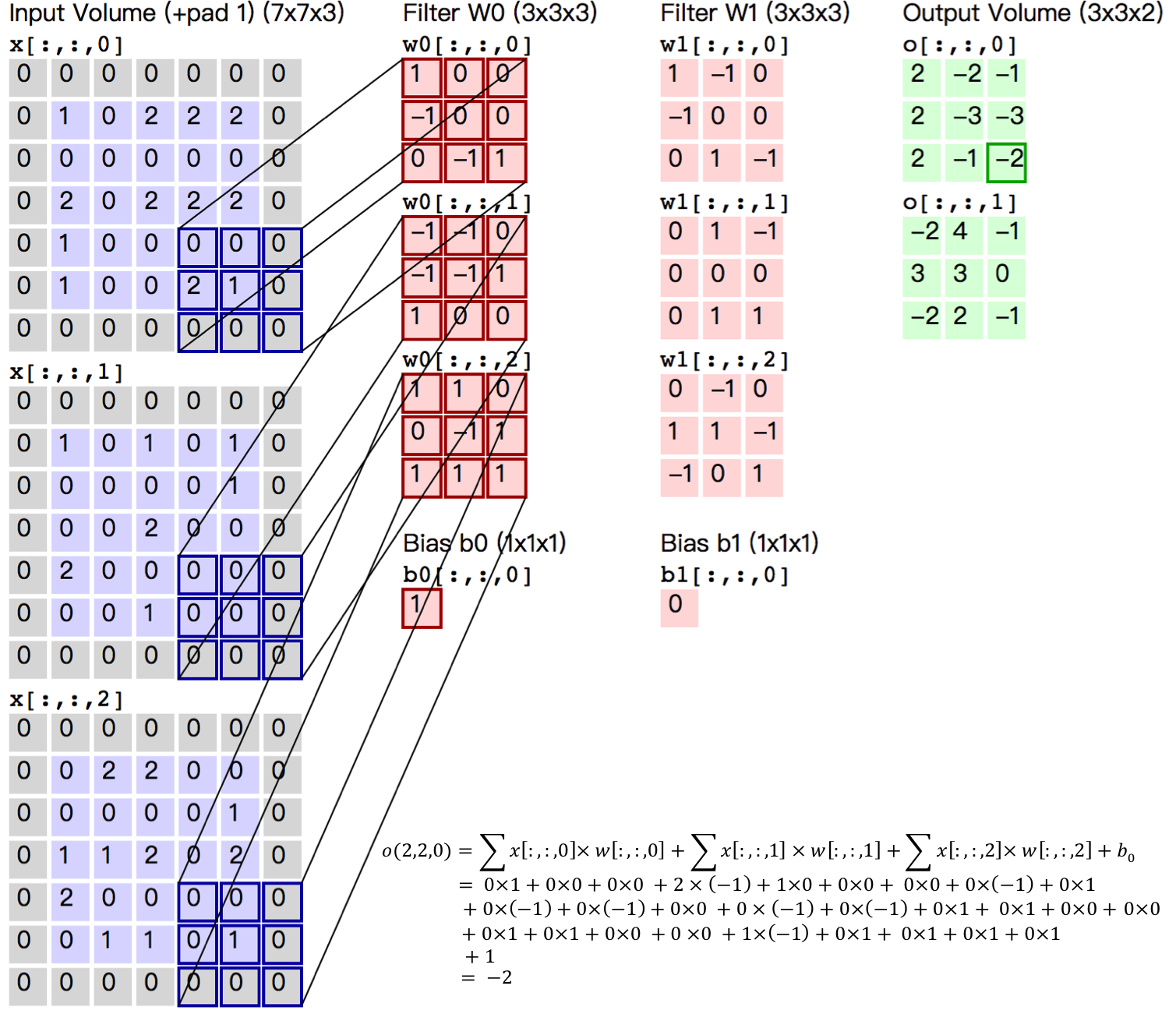• 局部连接：每个神经元仅与输入神经元的一块区域连接，这块局部区域称作感受野（receptive field）。在图像卷积操作中，即神经元在空间维度（spatial dimension，即上图示例H和W所在的平面）是局部连接，但在深度上是全部连接。对于二维图像本身而言，也是局部像素关联较强。这种局部连接保证了学习后的过滤器能够对于局部的输入特征有最强的响应。局部连接的思想，也是受启发于生物学里面的视觉系统结构，视觉皮层的神经元就是局部接受信息的。

• 权重共享：计算同一个深度切片的神经元时采用的滤波器是共享的。例如图5中计算$o[:,:,0]$的每个每个神经元的滤波器均相同，都为$W_0$，这样可以很大程度上减少参数。共享权重在一定程度上讲是有意义的，例如图片的底层边缘特征与特征在图中的具体位置无关。但是在一些场景中是无意的，比如输入的图片是人脸，眼睛和头发位于不同的位置，希望在不同的位置学到不同的特征 (参考斯坦福大学公开课)。请注意权重只是对于同一深度切片的神经元是共享的，在卷积层，通常采用多组卷积核提取不同特征，即对应不同深度切片的特征，不同深度切片的神经元权重是不共享。另外，偏重对同一深度切片的所有神经元都是共享的。

#### 池化层¶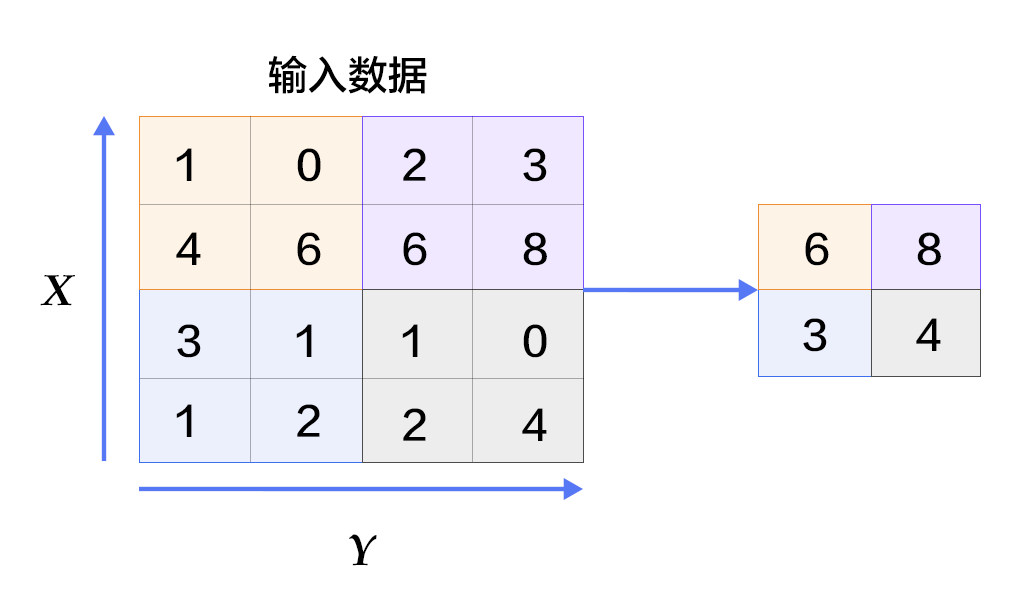### 常见激活函数介绍¶

• sigmoid激活函数：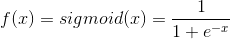• tanh激活函数：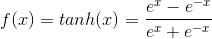• ReLU激活函数： $f(x) = max(0, x)$

## 数据介绍¶

PaddlePaddle在API中提供了自动加载MNIST数据的模块paddle.dataset.mnist。加载后的数据位于/home/username/.cache/paddle/dataset/mnist下：

train-images-idx3-ubyte 训练数据图片，60,000条数据
train-labels-idx1-ubyte 训练数据标签，60,000条数据
t10k-images-idx3-ubyte 测试数据图片，10,000条数据
t10k-labels-idx1-ubyte 测试数据标签，10,000条数据

## Fluid API 概述¶

1. inference_program：指定如何从数据输入中获得预测的函数， 这是指定网络流的地方。

2. train_program：指定如何从 inference_program标签值中获取 loss 的函数， 这是指定损失计算的地方。

3. optimizer_func: 指定优化器配置的函数，优化器负责减少损失并驱动训练，Paddle 支持多种不同的优化器。

## 配置说明¶

from __future__ import print_function # 将python3中的print特性导入当前版本
import os
from PIL import Image # 导入图像处理模块
import matplotlib.pyplot as plt
import numpy


### Program Functions 配置¶

• Softmax回归：只通过一层简单的以softmax为激活函数的全连接层，就可以得到分类的结果。

def softmax_regression():
"""
定义softmax分类器：
一个以softmax为激活函数的全连接层
Return:
predict_image -- 分类的结果
"""
# 输入的原始图像数据，大小为28*28*1
img = fluid.data(name='img', shape=[None, 1, 28, 28], dtype='float32')
# 以softmax为激活函数的全连接层，输出层的大小必须为数字的个数10
predict = fluid.layers.fc(
input=img, size=10, act='softmax')
return predict

• 多层感知器：下面代码实现了一个含有两个隐藏层（即全连接层）的多层感知器。其中两个隐藏层的激活函数均采用ReLU，输出层的激活函数用Softmax。

def multilayer_perceptron():
"""
定义多层感知机分类器：
含有两个隐藏层（全连接层）的多层感知器
其中前两个隐藏层的激活函数采用 ReLU，输出层的激活函数用 Softmax

Return:
predict_image -- 分类的结果
"""
# 输入的原始图像数据，大小为28*28*1
img = fluid.data(name='img', shape=[None, 1, 28, 28], dtype='float32')
# 第一个全连接层，激活函数为ReLU
hidden = fluid.layers.fc(input=img, size=200, act='relu')
# 第二个全连接层，激活函数为ReLU
hidden = fluid.layers.fc(input=hidden, size=200, act='relu')
# 以softmax为激活函数的全连接输出层，输出层的大小必须为数字的个数10
prediction = fluid.layers.fc(input=hidden, size=10, act='softmax')
return prediction

• 卷积池化层：在LeNet-5中会出现多个卷积-池化的操作，为避免代码重复书写，将串联的卷积-池化写成conv_pool函数。

def conv_pool(input, num_filters, filter_size, pool_size, pool_stride, act="relu"):
"""
定义卷积池化层：
含有一个卷积层和一个池化层
Args：
input —— 网络输入
num_filters —— 卷积核的个数
filter_size —— 卷积核的大小
pool_size —— 池化核的大小
pool_stride —— 池化的步长
act —— 卷积层的激活函数

Return:
out -- 经过卷积池化后的特征图
"""
conv_out = fluid.layers.conv2d(
input=input,
num_filters=num_filters,
filter_size=filter_size,
act=act)
out = fluid.layers.pool2d(
input=conv_out,
pool_size=pool_size,
pool_stride=pool_stride)
return out

• 卷积神经网络LeNet-5: 输入的二维图像，首先经过两次卷积层到池化层，再经过全连接层，最后使用以softmax为激活函数的全连接层作为输出层。

def convolutional_neural_network():
"""
定义卷积神经网络分类器：
输入的二维图像，经过两个卷积-池化层，使用以softmax为激活函数的全连接层作为输出层

Return:
predict -- 分类的结果
"""
# 输入的原始图像数据，大小为28*28*1
img = fluid.data(name='img', shape=[None, 1, 28, 28], dtype='float32')
# 第一个卷积-池化层
# 使用20个5*5的滤波器，池化大小为2，池化步长为2，激活函数为Relu
conv_pool_1 = conv_pool(
input=img,
filter_size=5,
num_filters=20,
pool_size=2,
pool_stride=2,
act="relu")
conv_pool_1 = fluid.layers.batch_norm(conv_pool_1)
# 第二个卷积-池化层
# 使用50个5*5的滤波器，池化大小为2，池化步长为2，激活函数为Relu
conv_pool_2 = conv_pool(
input=conv_pool_1,
filter_size=5,
num_filters=50,
pool_size=2,
pool_stride=2,
act="relu")
# 以softmax为激活函数的全连接输出层，输出层的大小必须为数字的个数10
prediction = fluid.layers.fc(input=conv_pool_2, size=10, act='softmax')
return prediction


#### Train Program 配置¶

def train_program():
"""
配置train_program

Return:
predict -- 分类的结果
avg_cost -- 平均损失
acc -- 分类的准确率

"""
# 标签层，名称为label,对应输入图片的类别标签
label = fluid.data(name='label', shape=[None, 1], dtype='int64')

# predict = softmax_regression() # 取消注释将使用 Softmax回归
# predict = multilayer_perceptron() # 取消注释将使用 多层感知器
predict = convolutional_neural_network() # 取消注释将使用 LeNet5卷积神经网络

# 使用类交叉熵函数计算predict和label之间的损失函数
cost = fluid.layers.cross_entropy(input=predict, label=label)
# 计算平均损失
avg_cost = fluid.layers.mean(cost)
# 计算分类准确率
acc = fluid.layers.accuracy(input=predict, label=label)
return predict, [avg_cost, acc]


#### Optimizer Function 配置¶

def optimizer_program():


### 数据集 Feeders 配置¶

batch是一个特殊的decorator，它的输入是一个reader，输出是一个batched reader。在PaddlePaddle里，一个reader每次yield一条训练数据，而一个batched reader每次yield一个minibatch。

# 一个minibatch中有64个数据
BATCH_SIZE = 64

batch_size=BATCH_SIZE)
# 读取测试集的数据，每次 yield 64个数据


### 构建训练过程¶

#### Event Handler 配置¶

event_handler 用来在训练过程中输出训练结果

def event_handler(pass_id, batch_id, cost):
# 打印训练的中间结果，训练轮次，batch数，损失函数
print("Pass %d, Batch %d, Cost %f" % (pass_id,batch_id, cost))

from paddle.utils.plot import Ploter

train_prompt = "Train cost"
test_prompt = "Test cost"
cost_ploter = Ploter(train_prompt, test_prompt)

# 将训练过程绘图表示
def event_handler_plot(ploter_title, step, cost):
cost_ploter.append(ploter_title, step, cost)
cost_ploter.plot()


event_handler_plot 可以用来在训练过程中画图如下：#### 开始训练¶

# 该模型运行在单个CPU上
use_cuda = False # 如想使用GPU，请设置为 True
place = fluid.CUDAPlace(0) if use_cuda else fluid.CPUPlace()

# 调用train_program 获取预测值，损失值，
prediction, [avg_loss, acc] = train_program()

# 输入的原始图像数据，名称为img，大小为28*28*1
# 标签层，名称为label,对应输入图片的类别标签
# 告知网络传入的数据分为两部分，第一部分是img值，第二部分是label值
feeder = fluid.DataFeeder(feed_list=['img', 'label'], place=place)

optimizer = optimizer_program()
optimizer.minimize(avg_loss)



PASS_NUM = 5 #训练5轮
epochs = [epoch_id for epoch_id in range(PASS_NUM)]

# 将模型参数存储在名为 save_dirname 的文件中
save_dirname = "recognize_digits.inference.model"

def train_test(train_test_program,

# 将分类准确率存储在acc_set中
acc_set = []
# 将平均损失存储在avg_loss_set中
avg_loss_set = []
acc_np, avg_loss_np = exe.run(
program=train_test_program,
feed=train_test_feed.feed(test_data),
fetch_list=[acc, avg_loss])
acc_set.append(float(acc_np))
avg_loss_set.append(float(avg_loss_np))
# 获得测试数据上的准确率和损失值
acc_val_mean = numpy.array(acc_set).mean()
avg_loss_val_mean = numpy.array(avg_loss_set).mean()
# 返回平均损失值，平均准确率
return avg_loss_val_mean, acc_val_mean


exe = fluid.Executor(place)
exe.run(fluid.default_startup_program())


main_program = fluid.default_main_program()
test_program = fluid.default_main_program().clone(for_test=True)


lists = []
step = 0
for epoch_id in epochs:
metrics = exe.run(main_program,
feed=feeder.feed(data),
fetch_list=[avg_loss, acc])
if step % 100 == 0: #每训练100次 打印一次log
print("Pass %d, Batch %d, Cost %f" % (step, epoch_id, metrics))
event_handler_plot(train_prompt, step, metrics)
step += 1

# 测试每个epoch的分类效果
avg_loss_val, acc_val = train_test(train_test_program=test_program,
train_test_feed=feeder)

print("Test with Epoch %d, avg_cost: %s, acc: %s" %(epoch_id, avg_loss_val, acc_val))
event_handler_plot(test_prompt, step, metrics)

lists.append((epoch_id, avg_loss_val, acc_val))

# 保存训练好的模型参数用于预测
if save_dirname is not None:
fluid.io.save_inference_model(save_dirname,
["img"], [prediction], exe,
model_filename=None,
params_filename=None)

# 选择效果最好的pass
best = sorted(lists, key=lambda list: float(list))
print('Best pass is %s, testing Avgcost is %s' % (best, best))
print('The classification accuracy is %.2f%%' % (float(best) * 100))


Pass表示训练轮次，Batch表示训练全量数据的次数，cost表示当前pass的损失值。

Pass 0, Batch 0, Cost 0.125650
Pass 100, Batch 0, Cost 0.161387
Pass 200, Batch 0, Cost 0.040036
Pass 300, Batch 0, Cost 0.023391
Pass 400, Batch 0, Cost 0.005856
Pass 500, Batch 0, Cost 0.003315
Pass 600, Batch 0, Cost 0.009977
Pass 700, Batch 0, Cost 0.020959
Pass 800, Batch 0, Cost 0.105560
Pass 900, Batch 0, Cost 0.239809
Test with Epoch 0, avg_cost: 0.053097883707459624, acc: 0.9822850318471338


## 应用模型¶

### 生成预测输入数据¶

infer_3.png 是数字 3 的一个示例图像。把它变成一个 numpy 数组以匹配数据feed格式。

def load_image(file):
# 读取图片文件，并将它转成灰度图
im = Image.open(file).convert('L')
# 将输入图片调整为 28*28 的高质量图
im = im.resize((28, 28), Image.ANTIALIAS)
# 将图片转换为numpy
im = numpy.array(im).reshape(1, 1, 28, 28).astype(numpy.float32)
# 对数据作归一化处理
im = im / 255.0 * 2.0 - 1.0
return im

cur_dir = os.getcwd()


### Inference 创建及预测¶

inference_scope = fluid.core.Scope()
with fluid.scope_guard(inference_scope):
# 使用 fluid.io.load_inference_model 获取 inference program desc,
# feed_target_names 用于指定需要传入网络的变量名
# fetch_targets 指定希望从网络中fetch出的变量名
[inference_program, feed_target_names,
save_dirname, exe, None, None)

# 将feed构建成字典 {feed_target_name: feed_target_data}
# 结果将包含一个与fetch_targets对应的数据列表
results = exe.run(inference_program,
feed={feed_target_names: tensor_img},
fetch_list=fetch_targets)
lab = numpy.argsort(results)

# 打印 infer_3.png 这张图片的预测结果
img=Image.open('image/infer_3.png')
plt.imshow(img)
print("Inference result of image/infer_3.png is: %d" % lab[-1])


## 参考文献¶

1. LeCun, Yann, Léon Bottou, Yoshua Bengio, and Patrick Haffner. "Gradient-based learning applied to document recognition." Proceedings of the IEEE 86, no. 11 (1998): 2278-2324.

2. Wejéus, Samuel. "A Neural Network Approach to Arbitrary SymbolRecognition on Modern Smartphones." (2014).

3. Decoste, Dennis, and Bernhard Schölkopf. "Training invariant support vector machines." Machine learning 46, no. 1-3 (2002): 161-190.

4. Simard, Patrice Y., David Steinkraus, and John C. Platt. "Best Practices for Convolutional Neural Networks Applied to Visual Document Analysis." In ICDAR, vol. 3, pp. 958-962. 2003.

5. Salakhutdinov, Ruslan, and Geoffrey E. Hinton. "Learning a Nonlinear Embedding by Preserving Class Neighbourhood Structure." In AISTATS, vol. 11. 2007.

6. Cireşan, Dan Claudiu, Ueli Meier, Luca Maria Gambardella, and Jürgen Schmidhuber. "Deep, big, simple neural nets for handwritten digit recognition." Neural computation 22, no. 12 (2010): 3207-3220.

7. Deng, Li, Michael L. Seltzer, Dong Yu, Alex Acero, Abdel-rahman Mohamed, and Geoffrey E. Hinton. "Binary coding of speech spectrograms using a deep auto-encoder." In Interspeech, pp. 1692-1695. 2010.

8. Kégl, Balázs, and Róbert Busa-Fekete. "Boosting products of base classifiers." In Proceedings of the 26th Annual International Conference on Machine Learning, pp. 497-504. ACM, 2009.

9. Rosenblatt, Frank. "The perceptron: A probabilistic model for information storage and organization in the brain." Psychological review 65, no. 6 (1958): 386.

10. Bishop, Christopher M. "Pattern recognition." Machine Learning 128 (2006): 1-58.